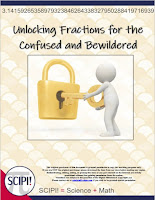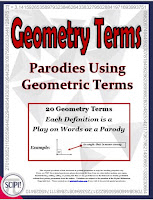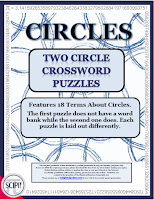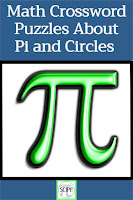### Unlocking Fractions for the Confused and Bewildered - A New Approach to Teaching FractionsI wish I understood this!
I teach remedial math on the college level, and I find that numerous students are left behind in the mathematical dust if only one strategy is used or introduced when learning fractions. Finding the lowest common denominator, changing denominators, not changing denominators, finding a reciprocal, and reducing to lowest terms are complex issues and often very difficult for many of my students.

I classify my students as mathphobics whose mathematical anxiety is hard to hide. One of my classes entitled, Fractions, Decimals and Percents, is geared for these undergraduates who have never grasped fractions. This article encompasses how I use a different method to teach adding fractions so these students can be successful. Specifically, let's look at adding fractions using the Cross Over Method.

Below is a typical fraction addition problem.  After writing the problem on the board, rewrite it with the common denominator of 6.
Procedure:

1) Ask the students if they see any way to multiply and make a 3 using only the numbers in this problem.

2) Now ask if there is a way to multiply and make 2 using just the numbers in the problem.

3) Finally, ask them to find a way to multiply the numbers in the problem to make 6 the denominator.

4) Instruct the students to cross their arms. This is the cross of cross over and means we do this by cross multiplying in the problem.

5) Multiply the 3 and 1, then write the answer in the numerator.  *Note: Always start with the right denominator or subtraction will not work.
6) Next multiply the 2 and 1 and write the answer in the numerator. Don’t forget to write the + sign. *Note: One line is drawn under both numbers. This is to prevent the students from adding the denominators (a very common mistake).7) Now have the students uncross their arms and point to the right using their right hand. This is the over part of cross over. It means to multiply the two denominators and write the product as the new denominator.

9) Reduce to lowest terms when necessary.\$4.50
It is important that students know the divisibility rules for 2, 3, 5, 6, 9 and 10. In this way, they can readily reduce any problem. In addition, it is extremely important that the students physically do the motions while they learn. This not only targets the kinesthetic learner but also gives the students something physical that makes the process easier to remember. The pictures or illustrations for each technique also benefit the visual/spatial learner. Of course, the auditory student listens and learns as you teach each method.

I have found these unconventional techniques are very effective for most of my students.  If you find this strategy something you might want to use in your classroom, a resource on how to add, subtract, multiply, and divide fractions is available by clicking the link under the resource cover. A video lesson is included to help you.

### Geometry Humor Can Make Mathematicians Smile

I 've been using Pinterest for as long as I can remember, and I love it. Not only do I post many resources and teaching ideas there, but I learn so-o-o much. For example, I learned how to pack one suitcase with enough stuff for a week. (My husband is thrilled with this one.) I also learned that when you fry bacon, to make a small cup out of aluminum foil; pour the bacon grease into it; let the grease harden; then close up the aluminum cup and toss it into the trash. That is one I use all of the time!

On my Pinterest account I have a board entitled Humor - We Need It! I post many math cartoons or humorous sayings there. My favorite subject to teach my college remedial math students is geometry, and I have plenty of corny jokes that I intersperse into my lessons. Here's one.

What did the little acorn say when it grew up? Gee- I'm - A - Tree! (Geometry)

What did the Pirate say when his parrot flew away? Polly-Gone (Polygon)

Here are some other geometry funnies from Pinterest.

Try placing a riddle or cartoon in the middle of a test.  I often do, and I know exactly where the students are by their laughs.  It helps them to relax and maybe get rid of those mathphobic tendencies.  I hope these math cartoons brought a smile to your face.  Have a great week of teaching!\$1.50
You might also like Geometry Parodies, a four page handout that includes 20 unusual definitions of geometry terms. Each definition is a play on words or a parody. Twenty-six geometric terms that are possible answers are listed in a word bank, but not all of the words are used in the matching exercise. An answer key is included.

### Does A Circle Have Sides?

Believe it or not, this was a question asked by a primary teacher.  I guess I shouldn't be surprised, but in retrospect, I was stunned. Therefore, I decided this topic would make a great blog post.

The answer is not as easy as it may seem. A circle could have one curved side depending on the definition of "side!"  It could have two sides - inside and outside; however this is mathematically irrelevant. Could a circle have infinite sides? Yes, if each side were very tiny. Finally, a circle could have no sides if a side is defined as a straight line. So which definition should a teacher use?

By definition a circle is a perfectly round 2-dimensional shape that has all of its points the same distance from the center. If asked then how many sides does it have, the question itself simply does not apply if "sides" has the same meaning as in a rectangle or square.I believe the word "side" should be restricted to polygons (two dimensional shapes). A good but straight forward definition of a polygon is a many sided shape.  A side is formed when two lines meet at a polygon vertex. Using this definition then allows us to say:

1) A circle is not a polygon.

2) A circle has no sides.

One way a primary teacher can help students learn some of the correct terminology of a circle is to use concrete ways.  For instance,  the perimeter of a circle is called the circumference.  It is the line that forms the outside edge of a circle or any closed curve. If you have a circle rug in your classroom, ask the students is to come and sit on the circumference of the circle. If you use this often, they will know, but better yet understand circumference.

For older students, you might want to try drawing a circle by putting a pin in a board. Then put a loop of string around the pin, and insert a pencil into the loop. Keeping the string stretched, the students can draw a circle!

And just because I knew you wanted to know, when we divide the circumference by the diameter we get 3.141592654... which is the number π (Pi)!  How cool is that?\$2.50
If you are studying circles in your classroom, you might like this resource. It is a set of two circle crossword puzzles that feature 18 terms associated with circles. The words showcased in both puzzles are arc, area, chord, circle, circumference, degrees, diameter, equidistant, perimeter, pi, radii, radius, secant, semicircle, tangent and two. The purpose of these puzzles is to have students practice, review, recognize and use correct geometric vocabulary in a fun, non-threatening way.

### Pi Day Is March 14th!

March 14 is Pi Day because March is the third month, and with 14 as the day, we get the first three digits of pi - 3.14! On Pi Day, nerds, geeks, and mildly interested geometry students alike come together and wear pi-themed clothing, read pi-themed books and watch pi-themed movies, all the while eating pi-themed pie.

Pi is an irrational number that approximately equals 3.14. It is the number you get if you divide the circumference of any circle by its diameter, and it's the same for all circles, no matter their size. You can estimate pi for yourself by taking some circular things like the tops of jars or round plates and measuring their diameter and their circumference. Then divide the circumference by the diameter, You should get an answer something like 3.14. It should be the same every time (unless you measured wrong).  In other words, π is the number of times a circle’s diameter will fit around its circumference

Actually, 3.14 is only approximately equal to pi. That's because pi is an irrational number. That means that when you write pi as a decimal it goes on forever and ever, never ending. (It is infinite.) Also, no number pattern ever repeats itself.

Usually in math, we write pi with the Greek letter π, which is the letter "p" in Greek. You pronounce it "pie", like the pie you eat for dessert. It is called pi because π is the first letter of the Greek word "perimetros" or perimeter.  What is interesting is that in the Greek alphabet, π (piwas) is the sixteenth letter; likewise, in the English alphabet, the letter "p" is also the sixteenth letter.

But hold your horses!  The fascination with pi isn't restricted to just mathematicians and scientists. Pi has a special place in popular culture, thanks to its frequency in mathematical formulas and its mysterious nature.  Even T.V. shows, books, and movies can’t help but mention π.

For example, pi gets mentioned in a scene from Twilight, in which vampire-boy Robert Pattinson recites the square root of pi.  In an episode of the Simpsons, two young girls at a school for the gifted play patty-cake and say “Cross my heart and hope to die, here’s the digits that make pi, 3. 1415926535897932384…”

Yep, whether you like it or not, pi is everywhere. Here are a few more places it has popped up:
1. The main character in the award-winning novel (and 2012 film) Life of Pi nicknames himself after π
2. A circular room in the Palais de la Découverte science museum in Paris is called the pi room. The room has 707 digits of pi inscribed on its wall. (The value of pi has now been calculated to more than two trillion digits.)
3. In an episode of Star Trek: The Original Series, Spock commands an evil computer to compute π to the last digit which it cannot do because, as Spock explains, “The value of pi is a transcendental figure without resolution.”
4. Pi is the secret code in Alfred Hitchcock’s Torn Curtain and in The Net starring Sandra Bullock.
Here is more arbitrary information related to pi that I found interesting.
1. If you were to print one billion decimal values of pi in an ordinary font, it would stretch from New York City to Kansas (where I live).
2.\$2.50
3.14 backwards looks like PIE.
3. "I prefer pi" is a palindrome. (It reads the same backwards as forwards)
4. Albert Einstein was born on Pi Day (March 14) in 1879.
All this information about pi and circles can be found in a Pi Day Crossword. It includes two different math crossword puzzles about Pi Day and features 20 words that have to do with pi or circles. One crossword includes a word bank which makes it easier to solve while the more challenging one does not. Even though the same vocabulary is used for each crossword, each grid is laid out differently. Answers keys for both puzzles are included.

By the way, notice my "handle" of Scipi.  The Sci is for science (what my husband teaches) and the pi is for π because I teach math.